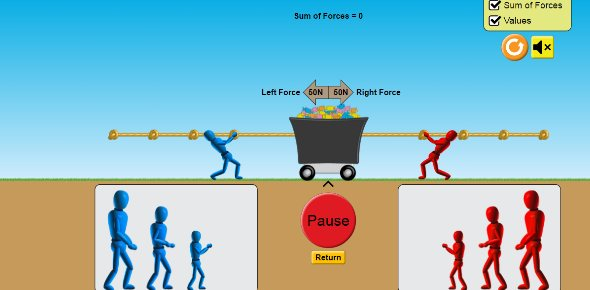# Additional: Force, Mass And Acceleration

10 Questions | Attempts: 1092SettingsA quiz on the basic points linking Force and Acceleration.

• 1.
If an object is moving at a constant velocity, the forces on it are:-
• A.

Unequal

• B.

Resolved

• C.

Forwards

• D.

Balanced

• E.

Opposite

• 2.
If there is a constant resultant force on an object the following will be happening:-
• A.

It's acceleration is increasing

• B.

It's acceleration is decreasing

• C.

It is moving with constant speed

• D.

It is moving with constant acceleration

• E.

It is stationary

• 3.
To cause an object that is moving to slow down you must:-
• A.

Balance the forces

• B.

Apply a resultant force in the direction of motion

• C.

Apply a resultant force in the opposite direction to the motion

• D.

Apply a resultant force at right angles to the motion

• E.

Make sure the forces are in equilibrium

• 4.
If a bicycle and rider of mass 80kg is accelerating at 1.5m/s2 the force pushing it is:-
• A.

0.02N

• B.

81.5N

• C.

120N

• D.

120m

• E.

120kg

• 5.
A lorry accelerates at 6m/s2 when the engine provides a resultant force of 48kN. What is the mass of the lorry?
• A.

133kg

• B.

28800kg

• C.

80kg

• D.

8000g

• E.

8000kg

• 6.
An aircraft of mass 4T gets 16kN of force from the engines. It's acceleration will be:-
• A.

64m/s2

• B.

4000m/s2

• C.

4m/s2

• D.

64000m/s2

• E.

250m/s2

• 7.
A motorcyclist of mass 250kg accelerates from rest to 20m/s in 4s. What is the force from the engine?
• A.

1250N

• B.

50N

• C.

1000N

• D.

5000N

• E.

20000N

• 8.
A seatbelt brings a passenger to a stop from 12m/s in just 2s. If the passenger has a mass of 60kg, what is the force exerted by the seatbelt?
• A.

720N

• B.

360N

• C.

10N

• D.

2.5N

• E.

0.25N

• 9.
Which of the following does NOT affect the thinking distance of a driver.
• A.

Drugs

• B.

Alcohol

• C.

Fog

• D.

Speed of the car

• E.

Tiredness

• 10.
Which of the following conditions have to be true when a parachutist reaches Terminal Velocity
• A.

The forces on her must be balanced

• B.

Her weight must be greater than air resistance

• C.

Her weight must be less than air resistance

• D.

Her speed must be greater than her weight

• E.

Her speed must be less than air resistance

## Related TopicsBack to top
×

Wait!
Here's an interesting quiz for you.